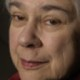# granddaughter’s first book

THE POOP MISTAKE – a book in progress

by Vimmy (age 6 years old)[final version to be] illustrated completely by Mom

^^^^^^^^^^^^^^^^^^^^^^^^^^^^^^^^^^^^^^^^^^^^^^^^^^^^^^^^^^^^^^^^^^

ONE DAY A FAMLIY GOT A DOG.

HIS NAME WAS KLAY.

HE WAS PLAYFUL–EXITED AND HAPPY.^^^^^^^^^^^^^^^^^^^^^^^^^^^^^^^^^^^^^^^^^^^^^^^^^^^^^^^^^^^^^^^^^^^^

THEN THEY ATE DINNER^^^^^^^^^^^^^^^^^^^^^^^^^^^^^^^^^^^^^^^^^^^^^^^^^^^^^^^^^^^^^^^^^^^^^^^^^^^^^^^^

THEN THEY TOOK A WALK. KLAY DID NOT NO HOW TO WALK ON A LESH,

SO HE PULLED AND PULLED AND HE PULLED.^^^^^^^^^^^^^^^^^^^^^^^^^^^^^^^^^^^^^^^^^^^^^^^^^^^^^^^^^^^^^^^^^^^^^^^^^^^^^^^^^^^^^

THE NEXT DAY THEY TOOK KLAY ON A WALK^^^^^^^^^^^^^^^^^^^^^^^^^^^^^^^^^^^^^^^^^^^^^^^^^^^^^^^^^^^^^^^^^^^^^^^^^^^^^^^^^^

AND WHEN THEY GOT TO THIER GRANDMA AND GRAMPA’S HOUS . . .

AFTER 20 MINITS HE POOPED ON THE RUG IN THER HOUS.^^^^^^^^^^^^^^^^^^^^^^^^^^^^^^^^^^^^^^^^^^^^^^^^^^^^^^^^^^^^^^^^^^^^^^^^^^^^^^^

AND EVERYONE WENT

NO!  NO!  NO!  NO!  NO!  NO!  NO!

^^^^^^^^^^^^^^^^^^^^^^^^^^^^^^^^^^^^^^^^^^^^^^^^^^^^^^^^^^^^^^^^^^^^^^^^^^^^^^

AND MOM SHUT HIM OUTSIDE WITH ME AND GRANDMA

[WHILE] MOM AND GRAMPA CLENED UP.^^^^^^^^^^^^^^^^^^^^^^^^^^^^^^^^^^^^^^^^^^^^^^^^^^^^^^^^^^^^^^^^^^^^^^^^^^^^^^^^

AND WHEN WE WERE COMING HOME

HE WAS BARKING AT APACHEY.^^^^^^^^^^^^^^^^^^^^^^^^^^^^^^^^^^^^^^^^^^^^^^^^^^^^^^^^^^^^^^^^^^^^^^^^^^^^^^^^^

WE LOVE KLAYBUT HE HAS A LOT TO LERN.

THE END

Notes from the editor:  Vimmy’s book was produced just as originally written. I didn’t have the opportunity to ask Klay (or Clay) how he preferred to spell his name, but Vimmy chose a K so I did it her way. She always like that. Exited means Klay was acting with “high energy.” Lesh is that thing attached to a dog’s collar, and Apachey (probably Apache) is an Airedale Terrior who lives in the neighborhood. I hope you enjoyed this “rough” draft  of Vimmy’s very first picture book as much as I’ve enjoyed posting it.

## 9 thoughts on “granddaughter’s first book”

1.Grace Roth on said:

Hooray for Vimmy!! Nobody could have written a better story. It has everything one would want in a well told tale!

2.grannymar on said:

Wonderful!

How about blurbing it? blurb.com

3.ruthek on said:

A charming book with great illustrations. I like it just as it is.

4.rbs on said:

LOVE IT! Including the creative spelling. Mom is an excellent artist, too!

5.mageb on said:

Dear Vimmy and Alice. Delightfully creative and well illustrated. The story leaves me grinning.

6.Raghu on said:

Awesome ! Loved it. I willl get Aish and Dyu to read and review it. 🙂

7.Raghu on said:

nice, really good for someone her age. She’s got talent!!!!!!:D

8.Raghu on said:

Wow! Vim’s gonna be a great writer one day, i’m sure 😀 -Aishma